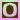Go to content
Use the mouse to put points in the blue area. After each point, the correlation coefficient and the regression equation will be calculated. To remove points, select the Erase point option, then click on the point you want removed. Clear plot will remove all the points; Residuals will pop up a scatter plot of X versus the residuals, as well as a histogram of the residuals. To add random points to the plot, press Random points, after changing the number and correlation for the new points if you wish.An applet from CUWUSTAT (regretfully, their web site appears to no longer be available).

## Glossary

### coefficient

The constant value in an expression, for example in 3x the coefficient of x is 3.

### correlation

The degree of relationship between two random variables, in the range -1 to 1.

### equation

A statement that two mathematical expressions are equal.

### histogram

A statistical diagram for continuous data similar to a bar chart but where the area of each bar is proportional to the frequency.

### plot

To mark a point on a graph accurately by using its coordinates.

### regression

A statistical model, whereby the expected value of a variable y is expressed in terms of known values of x.

### scatter plot

A scatter plot or scatter graph or scatter diagram displays as a collection of points, each having the value of one variable determining the position on the horizontal axis and the value of the other variable determining the position on the vertical axis.

Full Glossary List

## This question appears in the following syllabi:

SyllabusModuleSectionTopicExam Year
AQA A-Level (UK - Pre-2017)S1Correlation and RegressionCorrelation-
AQA AS Maths 2017StatisticsData Presentation and InterpretationCorrelation-
AQA AS/A2 Maths 2017StatisticsData Presentation and InterpretationCorrelation-
CCEA A-Level (NI)S2Correlation and RegressionCorrelation-
Edexcel A-Level (UK - Pre-2017)S1Correlation and RegressionCorrelation-
Edexcel AS Maths 2017StatisticsCorrelating Bivariate DataCorrelation-
Edexcel AS/A2 Maths 2017StatisticsCorrelating Bivariate DataCorrelation-
I.B. Higher Level7Correlation and RegressionCorrelation-
I.B. (MSSL)6Correlation and RegressionCorrelation-
OCR A-Level (UK - Pre-2017)S1Correlation and RegressionCorrelation-
OCR AS Maths 2017StatisticsScatter Diagrams and CorrelationCorrelation-
OCR MEI AS Maths 2017StatisticsScatter Diagrams and CorrelationCorrelation-
OCR-MEI A-Level (UK - Pre-2017)S2Correlation and RegressionCorrelation-
Pre-U A-Level (UK)ProbCorrelation and RegressionCorrelation-
Scottish (Highers + Advanced)HSCorrelation and RegressionCorrelation-